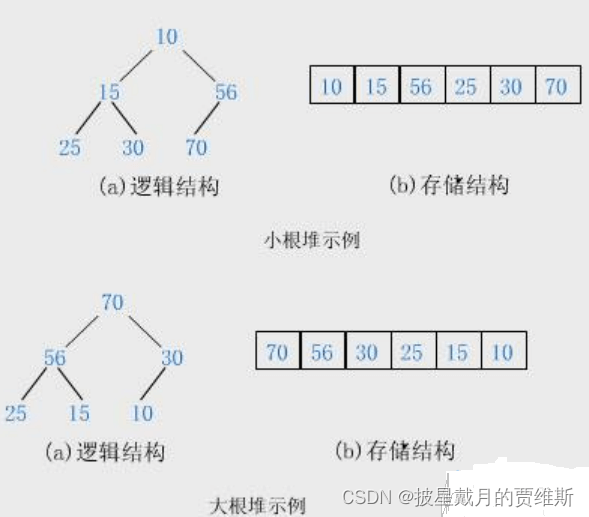﻿ C语言近万字为你讲透树与二叉树_C 语言_脚本之家
C 语言# C语言近万字为你讲透树与二叉树

## 一、树概念及结构

### 1.1 树的概念·有一个特殊的结点，称为根结点，根节点没有前驱结点

·除根节点外，其余结点被分成M(M>0)个互不相交的集合T1、T2、……、Tm，其中每一个集合Ti(1<= i <= m)又是一棵结构与树类似的子树。

·每棵子树的根结点有且只有一个前驱，可以有0个或多个后继 因此，树是递归定义的。### 1.2 树的相关概念### 1.3 树的表示## 二、二叉树概念及结构

### 2.1 概念

1. 或者为空

2. 由一个根节点加上两棵别称为左子树和右子树的二叉树组成1. 二叉树不存在度大于2的结点

2. 二叉树的子树有左右之分，次序不能颠倒，因此二叉树是有序树

### 2.2 特殊的二叉树：

1. 满二叉树：一个二叉树，如果每一个层的结点数都达到最大值，则这个二叉树就是满二叉树。也就是 说，如果一个二叉树的层数为K，且结点总数是 ，则它就是满二叉树。

2. 完全二叉树：完全二叉树是效率很高的数据结构，完全二叉树是由满二叉树而引出来的。对于深度为K 的，有n个结点的二叉树，当且仅当其每一个结点都与深度为K的满二叉树中编号从1至n的结点一一对 应时称之为完全二叉树。 要注意的是满二叉树是一种特殊的完全二叉树。

### 2.3 二叉树的性质

1. 若规定根节点的层数为1，则一棵非空二叉树的第i层上最多有 2^(i - 1)个结点.

2. 若规定根节点的层数为1，则深度为h的二叉树的最大结点数是2^h - 1.

3. 对任何一棵二叉树, 如果度为0其叶结点个数为n0 , 度为2的分支结点个数为n1 ,则有n0 ＝n2 ＋1.

4. 若规定根节点的层数为1，具有n个结点的满二叉树的深度，h=log2(n + 1) (是log以2 为底，n+1为对数)

5. 对于具有n个结点的完全二叉树，如果按照从上至下从左至右的数组顺序对所有节点从0开始编号，则对 于序号为i的结点有：

1. 若i>0，i位置节点的双亲序号：(i-1)/2；i=0，i为根节点编号，无双亲节点.

2.若2i+1<n, 左孩子序号；如果2i + 1 >=n 无左孩子

3. 若2i+2<n, 右孩子序号；如果2i + 1 >=n 无右孩子

### 2.4 二叉树的存储结构

#### 1. 顺序存储#### 2. 链式存储## 三、实现完全二叉树堆并实现堆排序

### 3.1 堆的概念和结构### 3.2 实现堆的难点

1、第一个数（根位置）和最后一个位置进行交换。

2、删除最后一个数据。

3、找出左右孩子中小的那个和父亲比较，如果比父亲小，交换，再从交换的孩子位置继续往下调整。```向下调整算法
void* AdjustDown(HPDataType* a, size_t size, size_t root)
{
size_t parent = root;
size_t child = parent * 2 + 1;//默认是左孩子
while (child < size)
{
//1、选出左右孩子中小的那个，而且保证不会越界访问
if (child + 1 < size && a[child + 1] < a[child])
{
child++;//左孩子变为右孩子
}
if (a[child]< a[parent])
{
Swap(&a[child], &a[parent]);
parent = child;//继续计算
child = parent * 2 + 1;//默认还是计算左孩子
}
else
{
break;
}
}
}```

```void Adjustup(HPDataType* a, size_t child)
{
size_t parent = (child - 1) / 2;
while (child > 0)
{
//小堆a[child] <a[parent],大堆a[child] > a[parent]时交换
if (a[child] < a[parent])
{
Swap(&a[child], &a[parent]);//因为是指针传参，所以要&
child = parent;
parent = (child - 1) / 2;
}
else
{
break;
}
}
}```

### 3.3 小堆的实现

```#pragma once
// 小堆
#include<stdio.h>
#include<assert.h>
#include<stdlib.h>
#include<stdbool.h>
typedef int HPDataType;
typedef struct Heap
{
HPDataType* a;
size_t size;
size_t capacity;
}HP;
void PrintTopk(int* a, int n, int k);
void HeapInit(HP* php);//堆的初始化
void HeapDestory(HP* php);//堆的销毁
void Swap(HPDataType* pa, HPDataType* pb);//交换
void HeapPrint(HP* php);
void AdjustDown(HPDataType* a, size_t size, size_t root);//堆的向下调整算法
//插入x以后，依旧保持堆
void HeapPush(HP* php, HPDataType x);
//删除堆顶的数据，（最小/最小）
void HeapPop(HP* php);
bool HeapEmpty(HP* php);
size_t HeapSize(HP* php);
HPDataType HeapTop(HP* php);```

```#include"Heap.h"
void HeapInit(HP* php)
{
assert(php);
php->a = NULL;
php->size = php->capacity = 0;
}
void HeapDestory(HP* php)
{
assert(php);
free(php->a);
php->a = NULL;
php->size = php->capacity = 0;
}
void Swap(HPDataType* pa, HPDataType* pb)
{
HPDataType tmp = *pa;
*pa = *pb;
*pb = tmp;
}
void HeapPrint(HP* php)
{
assert(php);
for (size_t i = 0; i < php->size; i++)
{
printf("%d ", php->a[i]);
}
printf("\n");
}
//涉及堆排序
{
size_t parent = (child - 1) / 2;
while (child > 0)
{
//小堆a[child] <a[parent],大堆a[child] > a[parent]时交换
if (a[child] < a[parent])
{
Swap(&a[child], &a[parent]);//因为是指针传参，所以要&
child = parent;
parent = (child - 1) / 2;
}
else
{
break;
}
}
}
void AdjustDown(HPDataType* a, size_t size, size_t root)
{
size_t parent = root;
size_t child = parent * 2 + 1;//默认是左孩子
while (child < size)
{
//1、选出左右孩子中小的那个，而且保证不会越界访问
if (child + 1 < size && a[child + 1] < a[child])//建大堆时< 改 >
{
++child;//左孩子变为右孩子
}
if (a[child] < a[parent])//建大堆时< 改 >
{
Swap(&a[child], &a[parent]);
parent = child;//继续计算
child = parent * 2 + 1;//默认还是计算左孩子
}
else
{
break;
}
}
}
void HeapPush(HP* php, HPDataType x)
{
assert(php);

if (php->size == php->capacity)//满了，需要扩容
{
size_t newCapacity = php->capacity == 0 ? 4 : php->capacity * 2;
//翻译：新定义一个无符号的newCapacity = 原来的capacity，如果原来的capacity = 0, 就赋值为4，4不够就再*二倍
HPDataType* tmp = realloc(php->a, sizeof(HPDataType)* newCapacity);
if (tmp == NULL)
{
printf("reallpc failed\n");
exit(-1);
}
php->a = tmp;
php->capacity = newCapacity;
}
//尾插
php->a[php->size] = x;
++php->size;
//向上调整保持是一个小堆
}
void HeapPop(HP* php)
{
assert(php);
assert(php->size > 0);
Swap(&php->a, &php->a[php->size - 1]);
--php->size;
}
bool HeapEmpty(HP* php)
{
assert(php);
return php->size == 0;
}
size_t HeapSize(HP* php)
{
return php->size;
}
HPDataType HeapTop(HP* php)
{
return php->a;
}```

### 3.4 堆的应用-堆排序

1. 建堆

2. 利用堆删除思想来进行排序 建堆和堆删除中都用到了向下调整，因此掌握了向下调整，就可以完成堆排序。

```void AdjustDown(HPDataType* a, size_t size, size_t root)
{
size_t parent = root;
size_t child = parent * 2 + 1;//默认是左孩子
while (child < size)
{
//1、选出左右孩子中小的那个，而且保证不会越界访问
if (child + 1 < size && a[child + 1] <a[child])//建大堆时< 改 >
{
++child;//左孩子变为右孩子
}
if (a[child] < a[parent])//建大堆时< 改 >
{
Swap(&a[child], &a[parent]);
parent = child;//继续计算
child = parent * 2 + 1;//默认还是计算左孩子
}
else
{
break;
}
}
}
void HeapSort2(int* a, int n)
{
//向上调整--建堆
//for (int i = 1; i < n; ++i)
//{
//}
//向下调整--建堆O（N）
for (int i = (n - 1 - 1) / 2; i >= 0; --i)
{
}
size_t end = n - 1;//n - 1是最后一个数据的下标
while (end > 0)
{
Swap(&a, &a[end]);
//次大的数到了倒数第二个位置
--end;
}
}
int main()
{
//	TestHeap();
int a[] = { 4 , 2, 7, 8, 5, 1, 0, 6 };
HeapSort(a, sizeof(a) / sizeof(int));
for (int i = 0; i < sizeof(a) / sizeof(int); i++)
{
printf("%d ", a[i]);
}
printf("\n");
return 0;
}```## 四.Top-k问题

TOP-K问题：即求数据结合中前K个最大的元素或者最小的元素，一般情况下数据量都比较大。

1. 用数据集合中前K个元素来建堆 前k个最大的元素，则建小堆 前k个最小的元素，则建大堆 2. 用剩余的N-K个元素依次与堆顶元素来比较，不满足则替换堆顶元素。```void PrintTopk(int* a, int n, int k)
{
//1、建堆-- 用a中前k个元素建堆
int* kminHeap = (int*)malloc(sizeof(int)* k);
assert(kminHeap);
//2、将剩余n-k元素依次与堆顶元素交换，不满则替换
for (int i = 0; i < k; i++)
{
kminHeap[i] = a[i];//将前k个数给给它
}
//第k-1个是最后一位数的下标，(k - 1 -1) / 2是倒数第一个非叶子结点
//建小堆
for (int j = (k - 1 - 1) / 2; j >= 0; --j)
{
}
//2、将剩余n - k元素依次与堆顶元素交换，不满则替换
for (int i = k; i < n; ++i)
{
if (a[i] > kminHeap)
{
kminHeap = a[i];
}
}
for (int j = 0; j < k; j++)
{
printf("%d ", kminHeap[j]);
}
printf("\n");
free(kminHeap);
}
void TestTopk()
{
int n = 10000;
int* a = (int*)malloc(sizeof(int)* n);
srand(time(0));
for (size_t i = 0; i < n; ++i)
{
a[i] = rand() % 1000000;
}
a = 1000000 + 1;
a = 1000000 + 2;
a = 1000000 + 3;
a = 1000000 + 4;
a = 1000000 + 5;
a = 1000000 + 6;
a = 1000000 + 7;
a = 1000000 + 8;
a = 1000000 + 9;
a = 1000000 + 1000;
PrintTopk(a, n, 10);
}
int main()
{
TestTopk();
return 0;
}```